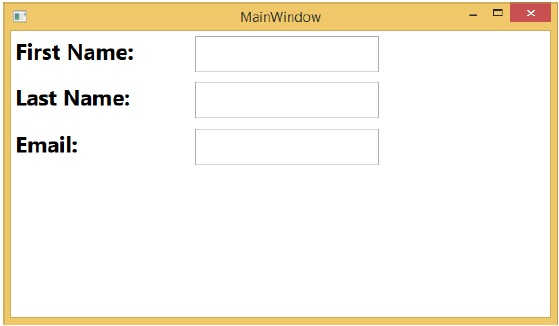# WPF - Application Level

Defining a style on app level can make it accessible throughout the entire application. Let’s take the same example, but here, we will put the styles in app.xaml file to make it accessible throughout application. Here is the XAML code in app.xaml.

```<Application x:Class = "Styles.App"
xmlns = "http://schemas.microsoft.com/winfx/2006/xaml/presentation"
xmlns:x = "http://schemas.microsoft.com/winfx/2006/xaml"
StartupUri = "MainWindow.xaml">

<Application.Resources>
<Style TargetType = "TextBlock">
<Setter Property = "FontSize" Value = "24" />
<Setter Property = "Margin" Value = "5" />
<Setter Property = "FontWeight" Value = "Bold" />
</Style>

<Style TargetType = "TextBox">
<Setter Property = "HorizontalAlignment" Value = "Left" />
<Setter Property = "FontSize" Value = "24" />
<Setter Property = "Margin" Value = "5" />
<Setter Property = "Width" Value = "200" />
<Setter Property = "Height" Value="40" />
</Style>

</Application.Resources>

</Application>
```

Here is the XAML code to create text blocks and text boxes.

```<Window x:Class = "Styles.MainWindow"
xmlns = "http://schemas.microsoft.com/winfx/2006/xaml/presentation"
xmlns:x = "http://schemas.microsoft.com/winfx/2006/xaml"
Title = "MainWindow" Height = "350" Width = "604">

<Grid>
<Grid.RowDefinitions>
<RowDefinition Height = "Auto" />
<RowDefinition Height = "Auto" />
<RowDefinition Height = "Auto" />
<RowDefinition Height = "*" />
</Grid.RowDefinitions>

<Grid.ColumnDefinitions>
<ColumnDefinition Width = "*" />
<ColumnDefinition Width = "2*" />
</Grid.ColumnDefinitions>

<TextBlock Text = "First Name: "/>
<TextBox Name = "FirstName" Grid.Column = "1" />
<TextBlock Text = "Last Name: " Grid.Row = "1" />
<TextBox Name = "LastName" Grid.Column = "1" Grid.Row = "1" />
<TextBlock Text = "Email: " Grid.Row = "2" />
<TextBox Name = "Email" Grid.Column = "1" Grid.Row = "2"/>
</Grid>

</Window>
```

When you compile and execute the above code, it will produce the following window.We recommend that you execute the above code and try to insert more features into it.

wpf_styles.htm